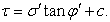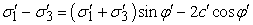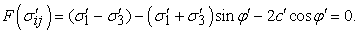﻿ Study of the Stability and Modeling by Finite Elements Using Plaxis 7.2<SUP>©</SUP> and Geoslope<SUP>©</SUP> of the Ramps A and J of the Toll Motorway at the site of “Patte d’oie” (Dakar-Senegal)Publications are Open
Access in this journal
Article Versions
Export Article
• Normal Style
• MLA Style
• APA Style
• Chicago Style
Research Article
Open Access Peer-reviewed

### Study of the Stability and Modeling by Finite Elements Using Plaxis 7.2© and Geoslope© of the Ramps A and J of the Toll Motorway at the site of “Patte d’oie” (Dakar-Senegal)

Oustasse Abdoulaye SALL , Makhaly BA, Déthié SARR
American Journal of Civil Engineering and Architecture. 2017, 5(4), 124-135. DOI: 10.12691/ajcea-5-4-1
Published online: August 17, 2017

### Abstract

This article focuses on the study of stability of embankments by finite element method of the straps A and J of the toll road of Dakar-Diamniadio on the section Malick SY-“Patte d’oie”. At the ramp A, deformations have amplitude of 301.93mm. Along AA’, the horizontal deformations are 222.5mm. This leads us to say that the deformations at this ramp are very important especially as allowable deformations in these kinds of structures are very small. Such deformations are larger in the horizontal direction of the embankment. The study shows that at the junction J, deformations are smaller and reach 91.99mm. The horizontal deflections according to section AA’ also show significant deformation equal to 87.63mm. This leads us to say like the above that these deformations are very important especially as these types of structures must withstand long so there have no impact at the road. The study of the stability on Géoslope of the straps A and J of the toll highway has allowed us to demonstrate and reinforce the idea that the slip lines are circular.

### 1. Introduction

Slope stability problems are frequently encountered in the construction of roads, canals, dikes and dams. Slope failure can be catastrophic and causes loss of life as well as considerable material damage. The estimate of the actual security with respect to the risk of rupture is complex, especially in the area of limited data.

A geotechnical model is built for stability calculations in which the topographic surface, the different layers of soil and hydraulic assumptions are set. These are essential and are a prerequisite for stability calculation. The distribution of pore pressure is important for the analysis of the mechanical stability.

The current stability analysis consists, on the basis of a two-dimensional description of the slope, to study the equilibrium conditions of a floor, supposedly monolithic, bounded by a failure surface and subject to the following efforts 1:

• Weight of massive,

• Reactions the ground up along the failure surface,

• Interstitial pressures ,

At a point of potential failure surface, the safety factor Fs (local) is defined as the ratio between the shear strength of the soil τmax and the shear stress τ actually exerted on the surface:

- If Fs > 1, there is no rupture,

- If Fs = 1, there is local failure.

The definition of this global safety factor faces two challenges: it is not known assessing except single cases or make complex modeling, the stress distribution along the failure surface and, if one has a such an assessment, it is generally observed that the safety factor is not constant along the fracture surface.

It is in this context that we were interested in studying the stability of embankments of straps A and J of the ‘‘patte d’oie’’ road (Senegal).

### 2. Presentation of the Project

Embankments of the straps A and J of the highway are large embankments that exceed ten feet (3 m), a study of their stability is necessary especially since they must have a strong stability that will allow them to resist long term. The proposed toll highway Dakar-Diamniadio is registered in the Urban Mobility Improvement Program (PAMU). The project has two phases:

• 1rst phase: Malick Sy - Pikine

The first phase consists of two lots, the first batch from the intersection “Malick Sy” to “Patte d'Oie” and a second batch from “Patte d'Oie” to Pikine. Our study was done in batch No1. It has a length of about 6.4 km. This project involves widening to dual three-lane including the redevelopment of all intersections.

2.1. Characteristics of Materials

Grain-size distribution, Atterberg limits and shear strength tests were conducted following ASTM D422, ASTM D4318, and ASTM D3080 respectively. The soil is a mixture of material made of clean sand found on the site and a sandy clay material from the excavations. The first material is clean sand which is a medium to fine sand of reddish color. It has good mechanical characteristic and lacking any swelling potential. This soil shows that 100% of the particles have a diameter greater than 2mm, 71.5 % to 99.5% of particles have a diameter less than 0.5mm, 0.7 % to 11.5 % have a diameter less than 0.1mm.

The second material is a clay from “Mamelle”. It is a material resulting from the alteration of volcanic formation of Dakar; it is in fact of basalt rocks. The study also showed that it has a plasticity index Ip of 33.9 % and a liquid limit WL of 61.8%. The results of the tests performed on the embankment soil are given in Table 1 to Table 4.

2.2. Calculation of Slope Stability and Finite Element Modeling

Plaxis© is a software that uses the finite element method and is particularly designed for the analysis of deformation and stability in geotechnical projects. Some geotechnical projects require advanced models for the study of nonlinear soil behavior simulation. Moreover, since the soil is subjected to multiple phases of requests, special procedures are required to treat the water. Although the definition of the floor model has undergone several major publications, many geotechnical engineering projects come up against the question of choosing type of model appropriate for the accurate determination of the interaction between structures and soil.

2.3. Soil Behavior Modeling

Soils and rocks behave nonlinearly under the effect of a load. This non- linear stress-strain behavior can be modeled according to different levels of accuracy which increases with the number of parameters introduced in the models. The Mohr-Coulomb model can be considered as a first order approximation of the actual behavior of the soil 4. This model, elastic perfectly plastic, incorporates five basic parameters which are:

ν : Poisson's ratio,

C: The cohesion;

φ: Angle of friction,

• Ψ : The dilatancy angle.

• Figure 1. Elastic behavior law perfectly plastic of Mohr -Coulomb type 

Several failure criteria have been established to describe the behavior of soils. Among them, the Mohr-Coulomb criterion (Figure 1) is the most used in the practice of geotechnical engineering to describe approximate way the behavior of granular soils (sand and gravel) and drained behavior long term soil saturated fine (silt and clay) 3.

The criterion of Mohr-Coulomb is shown schematically in plane stress (normal effective stress σ 'and tangential stress τ) by the following equation (Figure 1a):(1)

In terms of main effective stress (σ'1, σ'3), it can also be written (Figure 1b):(2)

with:

• σ’1, σ'3: major and minor principal effective stresses

• φ' : angle of internal friction

• c : cohesion.

The perfectly plastic linear elastic behavior with the criterion of Mohr-Coulomb (Figure 1b) is characterized by a linear elasticity (E, ν) and a yield stress, defined as cohesion c', the internal friction angle φ' in addition to the initial state.

We must make at least two triaxial tests at different confining pressures to determine the parameters of the model. The load surface F(σij) is expressed as follows:(3)

It is represented in the (σ', τ) by a straight line (Figure 1a).

### 3. Modeling Using Plaxis

Plaxis© allows for different types of finite element calculations. In practice, a project can be divided into several phases. Similarly, the process of Plaxis© calculation is also divided into calculation steps.

The activation of a predefined load case, the simulation of construction steps, introduction of a consolidation period, calculating a safety factor are examples of calculation phases. Each calculation phase is commonly divided into several steps of calculation.

This is due to the fact that the non-linear behavior of the soil requires application of the load by increments.

However, in most cases, it can be simply specifying the condition to get to the end of the calculation phase. In Plaxis©, automatic and robust procedures incrementing steps of loading provide a suitable choice of calculation steps. The dimensions of the transverse section of the embankment for each site are given in Figure 17 and Figure 19.

3.1. Presentation and Analysis of Results
3.1.1. Study of the Ramp A

The different results of the study of the deformation of the strap A are given in Figure 2 to Figure 8. At the junction A, we note deformations of 301.93mm. Along AA', we have horizontal deformations of 222.5mm. This leads us to say that the deformations are very important especially as allowable deformations in these kinds of structures are very small. At the base of the fill, we have deformations of 246.7mm. Such deformations are larger in the horizontal direction of the embankment. We note that for a load of 662 kPa (equivalent pressure of 13 tons corresponding to the value of the standard axle in Senegal). The ramp moves in two directions, horizontal and vertical. This can lead to a remarkable imbalance of the structure.

• Figure 2. Discretization of the medium (axial symmetry, plane stress and axial load 662 kN / m2)
• Figure 3. Long-term view of the deformation of the strap A
• Figure 4. Sense of long-term movements of the shoulder strap A
• Figure 5. Horizontal displacements of the structure following AA'
• Figure 6. Distortion of structure at the base of the embankment
• Figure 7. Effective pressure distribution through the sling A
• Figure 8. Effective pressure distribution across the ramp A along (AA')

3.1.2. Etude de la bretelle J

Table 2 below shows the values of the J ramp soil parameters used for modeling with Plaxis©.

The different results of the study of the deformation of the ramp J are given in Figure 9 to Figure 16. At the ramp J, we notice that the deformations are smaller and reach 91.99mm. The horizontal deflections according to section AA' also show significant deformation of 87.63mm. This leads us to say like the above that these deformations are very important especially as these types of structures must with stand long so there have no impact on the road.

• Figure 9. View through half a backfill J ramp with its stratigraphic log in addition to a load of 662 kN / m2 (AA')
• Figure 10. Distorted long term J ramp
• Figure 11. Direction of the long-term displacements of the ramp J
• Figure 12. Horizontal displacements of the ramp J (AA')
• Figure 13. Displacements of the ramp J along (BB') to the base of the embankment
• Figure 14. Overview of the actual pressure in the embankment of the ramp J
• Figure 15. Effective pressure following (AA')
• Figure 16. Effective pressure along (BB')

### 4. Determination of Critical Slip Lines and Calculating Safety Factors from Géoslope© Software

Géoslope is a software for the analysis of the stability of natural slopes or embankments. Checking the overall stability is carried out with the methods of the limit equilibrium.

The soil block subject to failure is divided into slices and for each one the program calculates the balance of forces: external forces, weight, forces acting at the base of each slice and shear forces acting along the interface the slices.

The equilibrium of the entire block of soil is the superposition of the equilibriums of each slice.

The failure surface is a sliding surface whose base is treated as a circular arc.

All points on the sliding surface have the same safety coefficient. The Géoslope© calculation code uses simplified methods of Bishop, Janbu and ordinary method.

Table 3 below shows the values of the parameters of ramp A used for modeling Géoslope©.

4.1. Result of the Analysis of the Ramps A and J on Géoslope©

The results for the study of the stability of embankment projects are given in Figure 17 to Figure 20.

• Figure 17. Géoslope© discretization of the ramp A
• Figure 18. Critical slip surface and safety factor of the ramp A
• Figure 19. Discretization Geoslope© of J ramp
• Figure 20. Critical slip surface and the safety factor of J ramp

Table 4 below shows the values of the ramp J parameters used for modeling in Géoslope©.

The study of the stability on Géoslope of the ramps A and J has allowed us to demonstrate and reinforce the idea that the slip lines are circular. This study also shows us that the two ramps presented satisfactory safety factors versus slip (safety factor Fs of 1.844 for ramp A and 2.001 for ramp J).

### 5. Conclusion and Recommendations

The results of this study lead to the following conclusions and remarks:

• Embankment ramps A and J, together, have an overall stability versus slip as shown with safety factors greater than 1.5. However, for such projects, a safety factor of 1.5 would be at the limit of stability, and that generally required Fs is greater than 2. This leads us to say that we should necessarily have a protection system of the slope.

• Study of the long-term behavior with Plaxis© are showed that embankments deform in both directions horizontal and vertical. The displacements obtained are very important and can cause a noticeable imbalance in the system. They are more important at the landfill body and at the slope.

• Significant subsidence in both cases. They are of the order of 30 cm which is the maximum thickness of a prescribed layer of sand so that it has a good compactness. These settlements, are often important sources of instability since it appears important to propose solutions to stabilize the long-term behavior of the embankment as:

- The introduction of resistance elements as a preventive measure to avoid trips, one consequence would be to reduce the shear strength of the soil. Compatibility of ground displacements and structures must be taken into account when selecting this type of technique.

- Building walls from prefabricated elements: walls prefabricated blocks, cell walls, embankments reinforced with geotextiles, metal or plastic frames, etc.

- Water penetration into the moving massive must be limited. The waters can come from sources zones, from a leak of waterproof, on a network and storm water. Indeed, surface water tends to seep into the cracks, to stagnate in areas of low slope and thus aggravate an initiated instability.

### References

  Blondeau F. (1976) les méthodes d’analyse de stabilité Bull. Labo. P et Ch., Spécial, p. 56-62. In article  Sall O. A (2015). Calcul analytique et modélisation de structure en plaque interaction sol-structure en vue du calcul des fondations superficielles en forme de radier- Thèse de doctorat de l’Université de Thiès, Sénégal, p. 125. In article  Pham P. T. Nguyen (2008). Etude en place et au laboratoire du comportement en petites déformations des sols argileux, Thèse de doctorat de l’École Nationale des Ponts et Chaussées, Paris, p. 226. In article View Article  Janbu N. (1963). Soil compressibility as determined by oedometer and triaxial tests. Proceedings of European Conference on Soil Mechanics and Foundation Engineering (ECSMFE), Wiesbaden, Vol. 1, p. 19-25. In articleThis work is licensed under a Creative Commons Attribution 4.0 International License. To view a copy of this license, visit http://creativecommons.org/licenses/by/4.0/